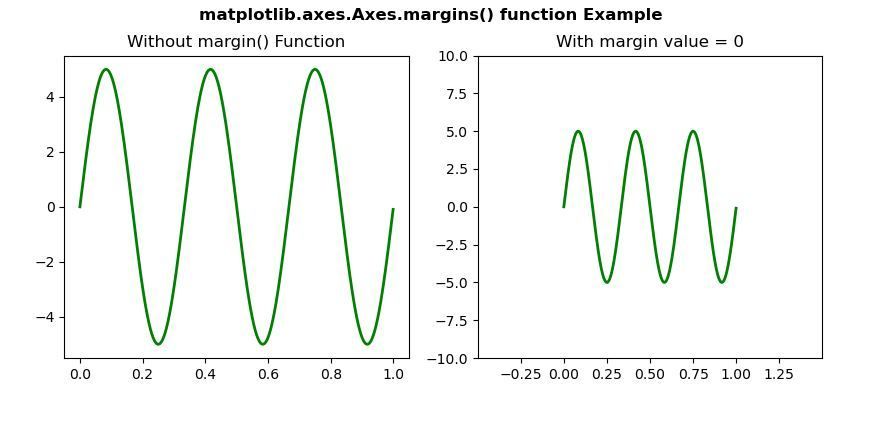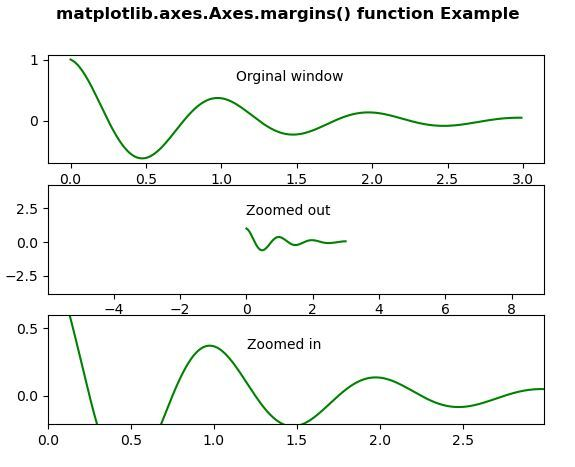Related Articles
Matplotlib.axes.Axes.margins() in Python
• Last Updated : 19 Apr, 2020

Matplotlib is a library in Python and it is numerical – mathematical extension for NumPy library. The Axes Class contains most of the figure elements: Axis, Tick, Line2D, Text, Polygon, etc., and sets the coordinate system. And the instances of Axes supports callbacks through a callbacks attribute.

## matplotlib.axes.Axes.margins() Function

The Axes.margins() function in axes module of matplotlib library is used to set or retrieve autoscaling margins.

Syntax: Axes.margins(self, *margins, x=None, y=None, tight=True)

Parameters: This method accepts the following parameters.

• *margins: This parameter is used to specify both margins of the x-axis and y-axis limits.
• x, y: These parameter is used to specific margin values for the x-axis and y-axis, respectively.
• tight : This parameter is passed to autoscale_view(), which is executed after a margin is changed.

Return value: The following values are returned by this method.

• xmargin
• ymargin

Below examples illustrate the matplotlib.axes.Axes.margins() function in matplotlib.axes:

Example 1:

 `# Implementation of matplotlib function  ``import` `numpy as np``import` `matplotlib.pyplot as plt``from` `matplotlib.widgets ``import` `Slider, Button, RadioButtons`` ` `fig, (ax, ax1) ``=` `plt.subplots(``1``, ``2``)``plt.subplots_adjust(bottom ``=` `0.25``)``t ``=` `np.arange(``0.0``, ``1.0``, ``0.001``)``a0 ``=` `5``f0 ``=` `3``delta_f ``=` `5.0``s ``=` `a0 ``*` `np.sin(``2` `*` `np.pi ``*` `f0 ``*` `t)``ax.plot(t, s, lw ``=` `2``, color ``=` `'green'``)`` ` `ax1.plot(t, s, lw ``=` `2``, color ``=` `'green'``)``ax1.margins(``0.5``)`` ` `ax.set_title(``"Without margin() Function"``)``ax1.set_title(``"With margin value = 0"``)`` ` `fig.suptitle('matplotlib.axes.Axes.margins() function \``Example\n', fontweight ``=``"bold"``)``fig.canvas.draw()``plt.show()`

Output:Example 2:

 `# Implementation of matplotlib function  ``import` `numpy as np``import` `matplotlib.pyplot as plt`` ` `t ``=` `np.arange(``0.0``, ``3.0``, ``0.01``)``t1 ``=` `np.exp(``-``t) ``*` `np.cos(``2` `*` `np.pi ``*` `t)`` ` `fig, [ax1, ax2, ax3] ``=` `plt.subplots(nrows ``=` `3``)``ax1.plot(t, t1, color ``=``"green"``)``ax1.text(``1.1``, ``0.65``, ``'Orginal window'``)`` ` `ax2.margins(``2``, ``2``)``ax2.plot(t, t1, color ``=``"green"``)``ax2.text(``0``, ``2.0``, ``'Zoomed out'``)`` ` `ax3.margins(x ``=` `0``, y ``=``-``0.25``)``ax3.plot(t, t1, color ``=``"green"``)``ax3.text(``1.2``, ``0.35``, ``'Zoomed in'``)`` ` `fig.suptitle('matplotlib.axes.Axes.margins() function\`` ``Example\n', fontweight ``=``"bold"``)``fig.canvas.draw()``plt.show()`

Output:Attention geek! Strengthen your foundations with the Python Programming Foundation Course and learn the basics.

To begin with, your interview preparations Enhance your Data Structures concepts with the Python DS Course. And to begin with your Machine Learning Journey, join the Machine Learning – Basic Level Course

My Personal Notes arrow_drop_up